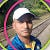# Java8: Lambdas & Functional Interfaces

Functional interfaces are type of interface which will have one method in it. Lambda expression facilitates functional programming, and simplifies the development. Functional interfaces can be used with lambdas as following.

If you have a close look at following example you will understand how a functional interface is used.

`public class FunctionalInterfaces {    public static void main(String[] args){        MyLambda fn = ()-> System.out.println("hello world");        fn.foo();    }}interface MyLambda{    void foo();}`

## Example 2

If you import java.util.function you will see many functional interfaces provided by java itself. Lets see how to use one of them.

`List<Integer> list= Arrays.asList(1, 2, 3, 4, 5, 6, 7, 8, 9);eval(list,n->n%2==0);`

In this example we will use predicate functional interface, will return boolean value based on provided implementation. In this example provided implementation is n->n%2==0, which will return true if the value is even.

`void eval(List<Integer> list, Predicate<Integer> predicate){    for(Integer n: list){        if(predicate.test(n)){            System.out.println(n+" ");        }    }}`

## Example 3

`public static void main(String[] args){    Calculator addCal=(a, b) -> a+b;    Calculator subCal=(a, b) -> a-b;    System.out.println(addCal.opertion(10,20));    System.out.println(subCal.opertion(20,30));}interface Calculator{    int opertion(int a, int b);}`

## Example 4

`List<Integer> list= Arrays.asList(1, 2, 3, 4, 5, 6, 7, 8, 9);System.out.println("Print  numbers: 3");eval2(list,n->n%2==0, n->System.out.println(n+" ") );`

In this example we are using consumer interface to pass the second command. compare with example 2.

`public static void eval2(List<Integer> list, Predicate<Integer>  predicate, Consumer consumer ){    for(Integer n: list){        if(predicate.test(n)){            consumer.accept(n);        }    }}`

--

--Sometimes A software Engineer, sometimes a support engineer, sometimes a devops engineer, sometimes a cloud engineer :D That’s how the this life goes!